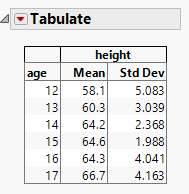Choose Language Hide Translation Bar
Highlighted

## Add formula column to tabulate table with JSL

Hi,

Is there some way to add formula column to tabulate table?

Formula column have to use final summary statistics.

For example, if I have Mean and StdDev columns, I would like to add a column of their sum (Mean * StdDev).

This is just example. My final goal is to add CP, CPK to tabulate...Thanks.

1 ACCEPTED SOLUTION

Accepted Solutions
Highlighted

## Re: Add formula column to tabulate table with JSL

JMP does not allow the adding of formula columns to Tabulate.  However, there are a few ways to get what you want.

1. Pre-summarize you data, adding in your example formula column of Mean*StdDev, then use Tabulate to display the summarized data using it's nice formating, etc.
2. Use the option under the red triangle in Tabulate to "Make into Data Table" and then in the data table add your new format column.
3. Use JSL an build your own Table Box(), which can get real close in replicating the Tabulate style output

Other Community Members may have other ideas too.

Jim
Highlighted

## Re: Add formula column to tabulate table with JSL

JMP does not allow the adding of formula columns to Tabulate.  However, there are a few ways to get what you want.

1. Pre-summarize you data, adding in your example formula column of Mean*StdDev, then use Tabulate to display the summarized data using it's nice formating, etc.
2. Use the option under the red triangle in Tabulate to "Make into Data Table" and then in the data table add your new format column.
3. Use JSL an build your own Table Box(), which can get real close in replicating the Tabulate style output

Other Community Members may have other ideas too.

Jim
Article Labels